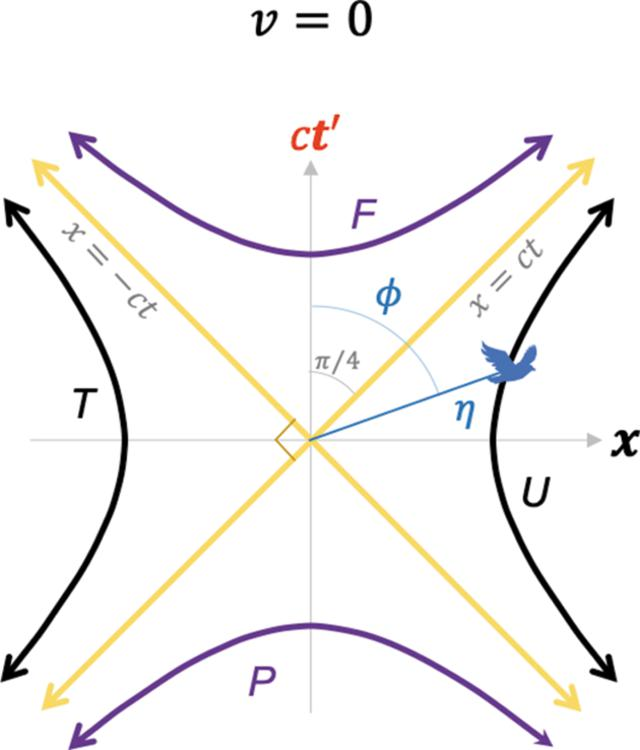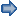disable zoom     view article Figure 4 A plot of the 2D Euclidean blended spacetime coordinates in equation (7a)with equation (8)substituted in it, for. The hyperbolas in Fig. 2are recovered but the angles are now Euclidean. For this case, the x andcoordinates are coincident (horizontal axis), and the ct andaxes are coincident (vertical axis), and. See the Mathematica script in the Mathematica notebook in the supporting information to generate this plot.FOUNDATIONSADVANCES
ISSN: 2053-2733
Volume 77| Part 4| July 2021| Pages 242-256